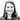# Post-Money-Valuation: Was ist die richtige Art, sie zu berechnen?

Die Post-Money-Valuation ist ein einfacher und gleichzeitig verwirrender Begriff, da jeder eine andere Formel zur Berechnung verwenden kann. In diesem Blogbeitrag diskutieren wir die verschiedenen Berechnungsmethoden!

Diese Seite ist nur verfügbar auf Englisch

Googling “post-money valuation” returns many results, some of them dive deep into the “accounting” explanations and others only acknowledge one calculation method. The reality is, that there is no industry-wide “right” way to calculate it.

However, there is an agreed-upon way. By agreed upon, we mean that you, a startup, agree on it with your lawyers and investors. As implied from this brief introduction, there are multiple methods for calculating it and we will explain and compare them in this post.

## 1. The first post-money valuation method

The first method is the most straightforward one, you add the value of the investment to the pre-money valuation of the company (post-money=pre-money + investment).

Sometimes, when calculating with this method, it’s not clear how to account for the pool shares.

1. If the pool existed before the new investor came in, then you could say it’s already included in the pre-money valuation.
2. Another way to include the pool in the calculation is to multiply the pool shares by the strike price and add it to the sum. The strike price can have a dramatic effect on the valuation. (If the strike price is high it may seem like artificial inflation of the company’s valuation.)

Pro-tip: A quick way to calculate post-money valuation is to take the investment amount and divide it by the expected ownership with that investment.

For example, Amazon would like to invest 2m for 20% ownership of your company. We can divide 2m by 20% and we get a 10m post-money valuation. Taking the first method as a way to calculate post-money valuation, we can now also calculate the pre-money valuation, by subtracting 2m from 10m.

#### 2. The second method

The second method takes into account the fully diluted number of shares and the share price. With this method, you can calculate the post-money valuation by multiplying the amount of fully diluted shares (incl. the pool) with the share price of the financing round.

#### The method Ledgy uses

At Ledgy we consulted with multiple law firms and venture funds and decided to calculate it by multiplying the fully diluted number of shares (incl. pools) and the share price of the round (modestly referred to as the “ledgy-way”).

There are two main reasons why this way of calculating makes sense:

1. As a shareholder, I expect that if I own X% of the fully diluted number of shares, it corresponds to X% of the post-money valuation. This property is only true if you calculate it the “ledgy-way”.
2. If there are shares that are purchased during the round with a discounted price (e.g. because of a convertible loan), then the method of simply saying post-money=pre-money+investment makes the company post-money valuation lower than its actual market value. This problem does not occur in the “ledgy-way”.

#### Which method is the industry standard?

As you can see there are a couple of ways to calculate the post-money valuation, and there is no industry standard. At the end of the day, what matters is how the post-money valuation is agreed upon and understood by the startup, its investors and lawyers.

Because we know this question is always present in the startup ecosystem, we decided to ask one of our investors for a little more clarification. The questions were answered by our investors from btov partners.

##### Which method of calculating post-money valuations do you use and why?

We do it with the following formula: post-money=pre-money+investment. With the other methods, one can argue that simply creating virtual shares for employees can’t increase the value of the company. If we used that method, the valuation would therefore always be 5-15% higher, depending on the size of the pool. Of course, this also influences the fund’s performance, each of our companies has employee stock ownership plans and the assets in our fund would be worth 1.05 - 1.15x more.

##### Have you seen other methods in use? By whom? (startup, lawyer, VC?)

We mostly noticed it in other funds, every accounting team calculates it differently.

##### Do you remember a situation where one party used a different method of calculation than the other? And did it cause any problems?

That's an exciting question! We recently had this as a discussion between different VCs because we had a clause in a portfolio company that said X would happen when the company raised the next round of financing with a post-money valuation > Ym EUR. Depending on the method of calculation, it was then above or below the threshold.